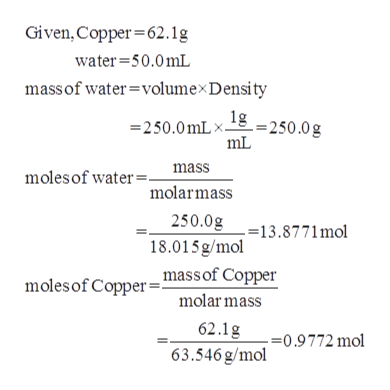# If a 62.1 gram piece of copper is heated to 100.0oC and then put into a vessel containing 250.0 mL of water at 1.5oC, what will be the final temperature of the water (in oC)?  Take the molar heat capacity of copper to be 24.4 J/(mol oC) and that of H2O(l) to be 75.3 J/(mol oC).  Assume the density of water is 1.00 g/mL.Your answer should have 3 significant figures.

Question
15 views

If a 62.1 gram piece of copper is heated to 100.0oC and then put into a vessel containing 250.0 mL of water at 1.5oC, what will be the final temperature of the water (in oC)?  Take the molar heat capacity of copper to be 24.4 J/(mol oC) and that of H2O(l) to be 75.3 J/(mol oC).  Assume the density of water is 1.00 g/mL.

check_circle

Step 1

The moles of copper and water...help_outlineImage TranscriptioncloseGiven, Copper 62.1g water 50.0mL massof water=volumex Density 250.0mLx 250.0g mL mass molesof water=. molarmass 250.0g 18.015 g/mol _mass of Copper -13.8771 mol molesof Copper molar mass 62.1g 63.546 g/mol .=0.9772 mol fullscreen

### Want to see the full answer?

See Solution

#### Want to see this answer and more?

Solutions are written by subject experts who are available 24/7. Questions are typically answered within 1 hour.*

See Solution
*Response times may vary by subject and question.
Tagged in

### Chemistry